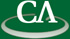### Algorithms for Additive and Projective Polynomials

#### Talk Slides

Thursday January 20th, 2011, at 11:00am, in K9509.

```Abstract:

The additive or linearized polynomials were introduced by Ore in 1933
as an analogy over finite fields to his theory of difference and
difference equations over function fields.  They have been employed in
number theory and algebraic geometry, and applied to constructing
error-correcting codes and cryptographic protocols.  In this talk we
will present fast algorithms for decomposing/factoring additive
polynomials. We will then look at the problem of counting the number
of right composition factors.  This is linked (algorithmically and
mathematically) to the number of rational roots of Abhyankar's
projective polynomials, for which we can get explicit counts.  We also
develop an inverse theory, counting the number of polynomials with a
given number of roots, or more generally, with a given Galois structure.
This is joint work with Joachim von zur Gathen and Konstantin Ziegler.
```